Click to Chat

1800-1023-196

+91-120-4616500

CART 0

• 0

MY CART (5)

Use Coupon: CART20 and get 20% off on all online Study Material

ITEM
DETAILS
MRP
DISCOUNT
FINAL PRICE
Total Price: Rs.

There are no items in this cart.
Continue Shopping• Complete Physics Course - Class 11
• OFFERED PRICE: Rs. 2,968
• View Details

```Velocity of wave in different media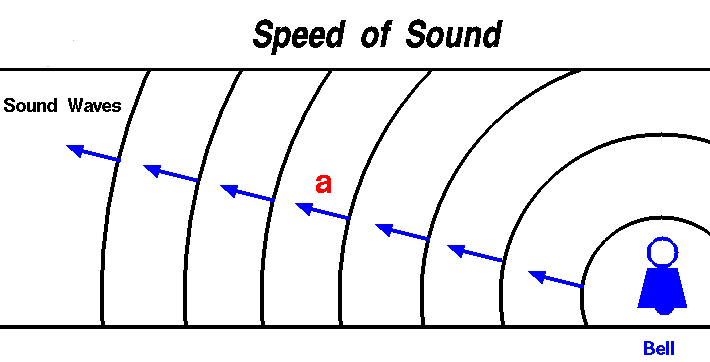The velocity of mechanical wave depends on elasticity and inertia of the medium.

Velocity of a transverse wave along a stretched string

Let us consider a string fixed at one of its ends and tension be applied at the other end. When the string is plucked at a point, it begins to vibrate.

Consider a transverse wave proceeding from left to right in the form of a pulse when the string is plucked at a point as shown in Fig. 7.4. EF is the displaced position of the string at an instant of time. It forms an arc of a circle with O as centre and R as radius. The arc EF subtends an angle 2θ at O.

If m is the mass per unit length of the string and dx is the length of the arc EF, then the mass of the portion of the string is m dx.

Centripetal force = (m) (dx) (v2)/R            …... (1)

This force is along CO. To find the resultant of the tension T at the points E and F, we resolve T into two components Tcos θ and T sinθ.

T cosθ components acting perpendi- cular to CO are of equal in magnitude but opposite in direction, they cancel each other.

T sin θ components act parallel  to CO. Therefore the resultant of the tensions acting at E and F is 2T sin θ. It is directed along CO. If θ is small, sinθ = θ and the resultant force due to tension is 2Tθ.

resultant force = 2Tθ

= 2T (dx/2R)         (Since, 2θ = dx/R)

= T (dx/R)                     …... (2)For the arc EF to be in equilibrium,

[(m) (dx) (v2)]/R = [(T) (dx)]/R

v2 = T/m

Or,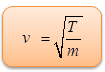…... (3)

Velocity of longitudinal waves in an elastic medium

Velocity of longitudinal waves in an elastic medium is…... (1)

where E is the modulus of elasticity, ρ is the density of the medium.

(i) In the case of a solid rod

v = √(q/ρ)           …... (2)

where q is the Young’s modulus of the material of the rod and ρ is the density of the rod.

(ii) In liquids, v = √(k/ρ)       …... (3)

where k is the Bulk modulus and ρ is the density of the liquid.

Newton’s formula for the velocity of sound waves in air

Newton assumed that sound waves travel through air under isothermal conditions (i.e) temperature of the medium remains constant.

The change in pressure and volume obeys Boyle’s law. ∴ PV = constant

Differentiating, P . dV + V .dP = 0

P. dV = –V dP

So, P = – dP/dV = change in pressure/volume strain

P = k (Volume Elasticity)

Therefore under isothermal condition, P = k

v = √(k/ρ) = √(p/ρ)

Here P is the pressure of air and ρ is the density of air. The above equation is known as Newton’s formula for the velocity of sound waves in a gas.

At NTP, P = 76 cm of mercury

= (0.76 × 13.6 × 103 × 9.8) N m–2 ρ = 1.293 kg m–3.

∴ Velocity of sound in air at NTP is,

v = √[0.76 × 13.6 × 103 × 9.8]/[1.293] = 280 ms-1

The experimental value for the velocity of sound in air is 332 m s–1. But the theoretical value of 280 m s–1 is 15% less than the experimental value. This discrepancy could not be explained by Newton’s formula.

Laplace’s correction

The above discrepancy between the observed and calculated values was explained by Laplace in 1816. Sound travels in air as a longitudinal wave. The wave motion is therefore, accompanied by compressions and rarefactions. At compressions the temperature of air rises and at rarefactions, due to expansion, the temperature decreases.

Air is a very poor conductor of heat. Hence at a compression, air cannot lose heat due to radiation and conduction. At a rarefaction it cannot gain heat, during the small interval of time. As a result, the temperature throughout the medium does not remain constant.

Laplace suggested that sound waves travel in air under adiabatic condition and not under isothermal condition.

For an adiabatic change, the relation between pressure and volume is given by,

P V γ = constant

Here, γ = Cp/Cv is the ratio of two specific haet capacities of the gas.

Differentiating,

PγVγ?-1.dV + Vγ?dP = 0

Pγ = –  Vγ?dP/Vγ?-1.dV

Pγ = – V (dP/dV)

Pγ = – dP/(dV/V) = k

So, Pγ = k (Volume elasticity)

velocity of sound, v = √(k/ρ) = √(γP/ρ)

This is Laplace’s corrected formula. For air at NTP

γ = 1.41, ρ = 1.293 kg m-3

So, v = √γ√P/ρ = √1.41×280 = 331.3 ms-1

This result agrees with the experimental value of 332 ms–1.

Factors affecting velocity of sound in gases(a)  Effect of pressure

If the temperature of the gas remains constant, then by Boyle’s law PV = constant

That is, P (m/ρ) = constant

p/ρ is a constant, when mass (m) of a gas is constant. If the pressure changes from P to P ′ then the corresponding density also will change from ρ to ρ ′ such that P/ρ is a constant.

In Laplace’s formula √(γP/ρ) is also a constant. Therefore the velocity of sound in a gas is independent of the change in pressure provided the temperature remains constant.

(b) Effect of temperature

For a gas, PV = RT

P (m/ρ) = RT

Or, P/ρ = RT/m

where m is the mass of the gas, T is the absolute temperature and R is the gas constant.

Therefore v = √γRT/m

It is clear that the velocity of sound in a gas is directly proportional to the square root of its absolute temperature.

Let vo and vt be the velocity of sound at Oo C and to C respectively. Then, from the above equation,

v0 = (√γR/m) (√273)

vt = (√γR/m) (√273+t)

So, vt/v0 = √[(273+t)/273]

Or, vt = v0[1+(t/273)]1/3

Using binomial expansion and neglecting higher powers we get,

vt =  v0[1+(1/2) (t/273)]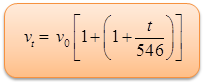Since,vo = 331 m s-1 at 0oC

vt = 331 + 0.61 m s–1

Thus the velocity of sound in air increases by 0.61 m s–1 per degree centigrade rise in temperature.

(c) Effect of density

Consider two different gases at the same temperature and pressure with different densities. The velocity of sound in two gases are given by,

v1 = √(γ1P/ρ1) and v2= √(γ2P/ρ2)

So, v1/v2 =√(γ1/γ2)(ρ2/ρ1)

For gases having same value of γ,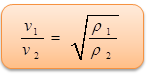The velocity of sound in a gas is inversely proportional to the square root of the density of the gas.

(d) Effect of humidity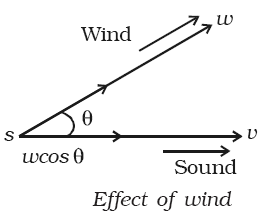When the humidity of air increases, the amount of water vapour present in it also increases and hence its density decreases, because the density of water vapour is less than that of dry air. Since velocity of sound is inversely proportional to the square root of density, the sound travels faster in moist air than in dry air. Due to this reason it can be observed that on a rainy day sound travels faster

(e) Effect of wind

The velocity of sound in air is affected by wind. If the wind blows with the velocity w along the direction of sound, then the velocity of sound increases to v + w. If the wind blows in the opposite direction to the direction of sound, then the velocity of sound decreases to v – w. If the wind blows at an angle θ with the direction of sound, the effective velocity of sound will be (v + w cos θ).

Note: In a medium, sound waves of different frequencies or wavelengths travel with the same velocity. Hence there is no effect of frequency on the velocity of sound.The propagation speeds of travelling waves are characteristic of the media in which they travel and are generally not dependent upon the other wave characteristics such as frequency, period, and amplitude.

The speed of sound in air and other gases, liquids and soilds is predictable from their density and elastic properties of the media.

The speed of sound in an ideal gas is independent of frequency, but does vary slightly with frequency in a real gas

Sound is a mechanical wave and travels by compression and rarefaction of the medium.

Light does not require a medium (one of the discoveries that mark the beginning of modern physics) in which to propagate.

Problem (JEE Main):

A transverse periodic wave on a string with a linear mass density of 0.200 kg/m is described by the following equation

y = 0.05 sin (420t – 21.0 x)

Here x and y are in metres and t is in seconds. Tension in the string is

(a) 32 N          (b) 42 N

(c) 66 N          (d) 80 N

Solution:

We know that, v = √T/m = ω/k

Since, T = m (ω/k)2

= (0.2) (420/21)2

= 80 N

From the above observation, we conclude that, option (d) is correct.Question 1

Which of the following does not affect the velocity of sound?

(a) temperature of the gas       (b) pressure of the gas

(c) mass of the gas                 (d) specific heat capacities of the gas

Question 2

When a wave passes from one medium to another, there is change of

(a) frequency and velocity

(b) frequency and wavelength

(c) wavelength and velocity

(d) frequency, wavelength and velocity

Question 3

Sound waves from a point source are propagating in all directions. What will be the ratio of amplitude at a distance 9 m and 25 m from the source?

(a) 25/9       (b) 9/25

(c) 3/5         (d) 81/625

Question 4

Sound of frequency 256 Hz passes through a medium. The maximum displacement is 0.1 m. The maximum velocity is equal to

(a) 60π m s-1             (b) 51.2π m s-1

(c) 256 m s-1              (d)  512 m s-1

Question 5

In a longitudinal wave there is state of maximum compression at a point at an instant. The frequency of wave is 50 Hz. After what time will the same point be in the state of maximum rarefaction.

(a) 0.01 s       (b) 0.002 s

(c) 25 s          (d) 50 sQ.1
Q.2
Q.3
Q.4
Q.5

b

c

a

b

a

Related Resources:-

You might like to refer mechanical wave motion.

For getting an idea of the type of questions asked, refer the  Previous Year Question Papers.

To read more, Buy study material of Waves comprising study notes, revision notes, video lectures, previous year solved questions etc. Also browse for more study materials on Physics here.
```### Course Features

• 101 Video Lectures
• Revision Notes
• Previous Year Papers
• Mind Map
• Study Planner
• NCERT Solutions
• Discussion Forum
• Test paper with Video Solution